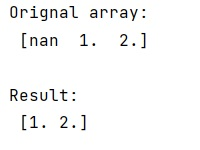# 'isnotnan' functionality in numpy, can this be more pythonic?

Learn whether is there any functionality in numpy to check if a value is not a nan value?
Submitted by Pranit Sharma, on January 11, 2023

NumPy is an abbreviated form of Numerical Python. It is used for different types of scientific operations in python. Numpy is a vast library in python which is used for almost every kind of scientific or mathematical operation. It is itself an array which is a collection of various methods and functions for processing the arrays.

## 'isnotnan' functionality in numpy

While creating a DataFrame or importing a CSV file, there could be some NaN values in the cells. NaN values mean "Not a Number" which generally means that there are some missing values in the cell.

To check if a value is not a nan value, we will use isnan() method along with tilde(~) operator.

The tilde sign (~) in pandas is used when we work with Boolean values. In programming, we sometimes use some specific values that only have two values, either True or False. These values are known as Boolean values.

This tilde sign works as a negation operator that means is used to reverse the Boolean values.

Let us understand with the help of an example,

## Python code to demonstrate the example of 'isnotnan' functionality in numpy

```# Import numpy
import numpy as np

# Creating numpy array
arr = np.array([np.nan, 1, 2])

# Display original array
print("Orignal array:\n",arr,"\n")

# Check for each value
res = arr[~np.isnan(arr)]

# Display result
print("Result:\n",res,"\n")
```

Output:What's New (MCQs)

Top Interview Coding Problems/Challenges!

IncludeHelp's Blogs

Languages: » C » C++ » C++ STL » Java » Data Structure » C#.Net » Android » Kotlin » SQL
Web Technologies: » PHP » Python » JavaScript » CSS » Ajax » Node.js » Web programming/HTML
Solved programs: » C » C++ » DS » Java » C#
Aptitude que. & ans.: » C » C++ » Java » DBMS
Interview que. & ans.: » C » Embedded C » Java » SEO » HR
CS Subjects: » CS Basics » O.S. » Networks » DBMS » Embedded Systems » Cloud Computing
» Machine learning » CS Organizations » Linux » DOS
More: » Articles » Puzzles » News/Updates